# B-spline Curves: Modifying KnotsBecause a B-spline curve is the composition of a number of curve segments, each of which is defined on a knot span, modifying the position of one or more knots will change the association between curve segments and knot spans and hence change the shape of the curve.

The following figures depict the effect of modifying a single knot. It is a B-spline curve of degree 6 with 17 knots with the first seven and last seven clamped at the end points, while the internal knots are 0.25, 0.5 and 0.75. The initial curve is shown in the left. If knot 0.25 is moved to 0.1, the shape of the curve changes and the original C(0.25) moves downward to a new position. If knot 0.5 is moved to 0.1 so that knot 0.1 becomes a double knot (with multiplicity 2), the shape of the curve moves to the left; but C(0.1) is moved upward to a position near to the original point (i.e., the original C(0.25)). The result is shown in the right figure. Moreover, even though we have a double knot at 0.1 and another knot at 0.75 that unevenly subdivide the domain [0,1] into three knot spans, the B-spline curve is more or less evenly subdivided by their corresponding points.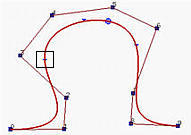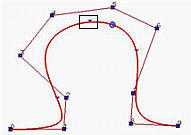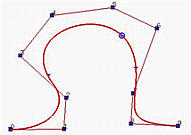The following figure shows the change of shape of three curves, each of which is defined by 10 (n=9) control points and is of degree 6. Their internal knot vectors are (0.25,0.5,0.75) - red curve, (0.25,0.25,0.75) - blue curve, and (0.25,0.25,0.25) - black curve.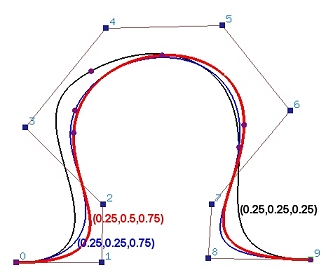Practical experience shows that modifying knot positions is neither predictable nor satisfactory. More precisely, because it is not clear how the shape of a B-spline curve will respond to the change of the knot vector, modifying the shape of B-spline curve by changing knots is usually unsatisfactory and difficult to achieve the desired goal.### A Note about Multiple Knots

Multiple knots may help to generate expect/desirable results. Recall from a property of multiple knots discussed earlier that increasing the multiplicity of an internal knot decreases the number of non-zero basis functions at this knot. In fact, if the multiplicity of this knot is k, there are at most p - k + 1 non-zero functions at this knot. Moreover, the basis functions are Cp-k continuous at this knot.

Suppose a knot has multiplicity p-k, there will be k+1 non-zero basis functions at this knot and the corresponding point on the curve lies in the convex hull defined by the control points that correspond to these non-zero basis functions. If k = p - 1, there are two non-zero functions and the corresponding convex hull is a line segment.

If k = p, there is only one non-zero basis function at this knot and there is only one control point has non-zero coefficient. As a result, the curve passes through this point!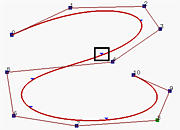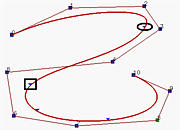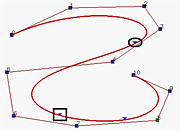(a) (b) (c)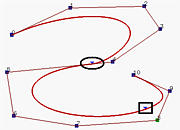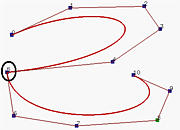(d) (e)

In Figure (a) above, which shows a B-spline curve of degree 5, the knot that corresponds to the marked knot point is moved to its previous knot, creating a knot of multiplicity two. The result is in Figure (b), which does not show much difference from the original. Then, the next knot (marked with a rectangle) is moved to the resulting knot (marked with an ellipse) again, creating a knot of multiplicity three. This is in Figure (c). The resulting curve moves towards a leg of the control polyline. Moving one more knot creates a new knot of multiplicity four. This forces the corresponding point (marked with an ellipse) lies on a leg. Finally, the only remaining knot is moved to combined with the other knots. Since its multiplicity is five and p=5, there is only one non-zero coefficient and hence forces the curve to pass that control point. As shown in Figure (e), that control point is P5.